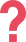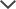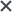## ISEE Sample Questions

If you are looking for additional Verbal Reasoning practice questions, try our full prep packs below!
In the ISEE exam, there are 40 questions in this section. Our prep packs contain 75+ Verbal Reasoning questions, so you can master the verbal section.

 Question 3: Quantitative Reasoning Question If, which expression is equal to 𝑥? A) y-2 B) 5(y-10) C) (y-10)/5 D) (y+10)/5 Answer & Explanation ▼ | ▲ The correct answer is (C). To solve this problem, isolate the desired variable, 𝑥. To do so, put every term that contains the variable on one side of the equation and every term that does not contain the variable on the other side: y-10=5x Now, divide both sides of the equation by the coefficient of 𝑥, which is 5: (y-10)/5 = 𝑥 Thus, the value of 𝑥 is (y-10)/5 , and the correct answer is (C).

 Question 4: Quantitative Reasoning Question In a large company, there are five sales representatives. In a certain week, their sales are as follows:\$1100, \$1105, \$1107, \$1112, and \$1156. What are the average sales per person for that week? A) \$1107 B) \$1114 C) \$1116 D) \$1128 Answer & Explanation ▼ | ▲ The correct answer is (C). To solve this problem, find the average of the five values given. Note that all values can be represented as \$1100 + a two-digit number, thus we can calculate the average for the two-digit numbers, and conclude for the original values. (When calculating an average, find the sum of the values, and divide the sum by the number of values. If you were to calculate the average with the original values, the result would be 5500 + the sum of the two-digit numbers. Divide this by 5 - the number of values - and get 1100 + the average of the five two-digit numbers.) Calculate the sum of 00, 05, 07, 12, and 56: 0 + 5 + 7 + 12 + 56 = 80 Divide the sum by 5 (80 ÷ 5 = 16). Add the missing 1100 and conclude that the average sale for that week is \$1116, and the correct answer is (C).

We have a variety of Quantitative Reasoning questions in our full prep packs below!
The ISEE test contains 37 Quantitative Reasoning questions. Our prep packs include 70+ Quantitative Reasoning questions, and much more, starting from just 59\$

 Question 5: Mathematics Achievement Question If (3.75 + 1.25)𝑎 = (11.03 − 1.03)𝑎, what is the value of 𝑎? A) 0 B) 0.5 C) 5 D) 10 Answer & Explanation ▼ | ▲ The correct answer is A. In this problem, since 3.75 + 1.25 = 5, the left side of the equation equals 5𝑎. Since 11.03 − 1.03 = 10, the right side of the equation equals 10𝑎. The result is: 5𝑎 = 10𝑎. Assuming that 𝑎 ≠ 0, divide both sides of the equation by 𝑎, and conclude that 5 = 10, which is a false equation. Thus, the only case in which 5𝑎 = 10𝑎 is when 𝑎 = 0, and then 5 × 0 = 10 × 0 = 0, and the correct answer is A.
 Question 6: Mathematics Achievement Question A bag contains 30 marbles, colored red, blue, and yellow. For every two red marbles there are 3 blue marbles. There are five yellow marbles. How many blue marbles are there in the bag? A) 5 B) 10 C) 12 D) 15 Answer & Explanation ▼ | ▲ he correct answer is (D). In this problem, find the number of blue marbles, given a certain ratio. There are five yellow marbles, therefore, there are 30 – 5 = 25 red and blue marbles. You know that for every two red marbles, there are three blue marbles. Thus, the ratio of red marbles to blue marbles is 2:3, and you can write 2𝑥 + 3𝑥 = 25. 5𝑥 = 25 𝑥 = 5 3𝑥 = 15 One may conclude that there are 15 blue marbles in the bag, and the correct answer is (D).

Use our full prep packs below if you need more practice in the Mathematics Achievement section           The ISEE exam contains 47 questions. Our comprehensive preparation includes-90+ Mathematics achievement questions, so when testing day comes you will be stress-free and ready.

 Question 8: Reading Comprehension Question Read the paragraph and answer the question. 1 In the wild, packs of animals are usually arranged in a strict hierarchy. In most animal 2 species, the young are usually found to be at the lower levels, followed by the females, while the 3 males are highest up. Yet, within the males there are also ranks. For instance, when observing a 4 pride of lions, it promptly becomes clear that one male is feared by all the others. In line 4, the word "pride" most nearly means- A) leader. B) Pack C) Ranking. D) Wild. Answer & Explanation ▼ | ▲ The correct answer is (B). Answer (B) is the correct answer because a pack of lions is called a pride. The sentence refers to observing a pack of lions. Answers (A), (C), and (D) are incorrect because they have other meanings. In addition, they can be eliminated because they do not construct a logical sentence.

If you need more practice in the Reading Comprehension section, just click below!
The ISEE test contains 36 questions in this section. Our detailed prep pack includes over 70 practice questions of the Reading Comprehension section.

## ISEE Practice Tests from TestPrep-Online

Access our full ISEE practice packs now, with full-length tests including hundreds of practice questions and answer explanations.

 Free ISEE Practice Resources Full ISEE PrepPacks ISEE Middle Sample Test ISEE Middle Full Pack ISEE Upper Sample Test ISEE Upper Full Pack

## What is the ISEE Test?

Watch the following video to learn everything you need to know about the ISEE test and how to score high on it. Understand the structure and format of the ISEE, try sample questions, and get important tips for acing this test.## Our Online Services

TestPrep-Online offers a unique, online testing experience. All of our test prep material is available online, giving you and your child the freedom to study whenever and wherever you want. Our test packages offer a user-friendly interface, which enables you to easily navigate through a multitude of practice tests, video tutorials, study guides, additional drills, and example test questions. Prepare your child from the comfort of your own home, without the added cost and hassle of a tutoring service.

The ISEE and other trademarks are the property of their respective trademark holders. None of the trademark holders are affiliated with TestPrep-Online or this website.

Need HelpNeed Help